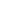# Ancient Mathematics: China

Ancient Chinese measurements with triangle and compass were already performed in the 20th c BCE. In “The Nine Chapters on the Mathematical Art” (around 200 BCE), Chinese mathematicians performed calculations with fractions, square roots and geometrical progressions. They found areas and volumes using 3 as an approximation for PI, and solved systems of linear equations using negative numbers. They knew a method of finding triangular triples (Pythagoras triples). Later, in the 5th c CE, they calculated PI with great accuracy. They discovered binominal coefficients known to Europeans as Pascal’s triangle two centuries before Pascal.

• Face-to-Face

#### SUGGESTED READING

• Jean-Claude Martzloff. A History of Chinese Mathematics. Springer, 1997.

#### COURSE OUTLINE

• Chronology and some of the significant persons in the history of Chinese mathematics
• Magic square by Lo Shu (650 BCE)
• “The Nine Chapters on the Mathematical Art” (around 200 BCE)
• Calculations with fractions, areas and volumes
• Systems of linear equations and calculations of square triangles (Pythagoras theorem)
• Calculation of triangular triples (Pythagorean triples)
• Distance to inaccessible objects
• Approximations for PI
• Chinese trigonometry
• Binominal coefficients by Yang Hui

#### PLANNED LEARNING OUTCOMES

By the end of this course, students should be able to:

1. Understand ancient Chinese foundations of mathematics.
2. Become acquainted with the Ancient Chinese numerals.
3. Know achievements of “The Nine Chapters on the Mathematical Art”.
4. Become acquainted with Chinese trigonometry.
5. Know Yang Hui’s introduction to binominal coefficients (Pascal triangle).
\$65 Limited

<p>Ancient Chinese measurements with triangle and compass were already performed in the 20th c BCE. In “The Nine Chapters on the Mathematical Art” (around 200 BCE), Chinese mathematicians performed

...
16 Jul
Mo Tu We Th Fr Sa SuInterested in this course but can't attend? Please join the waiting list by clicking .